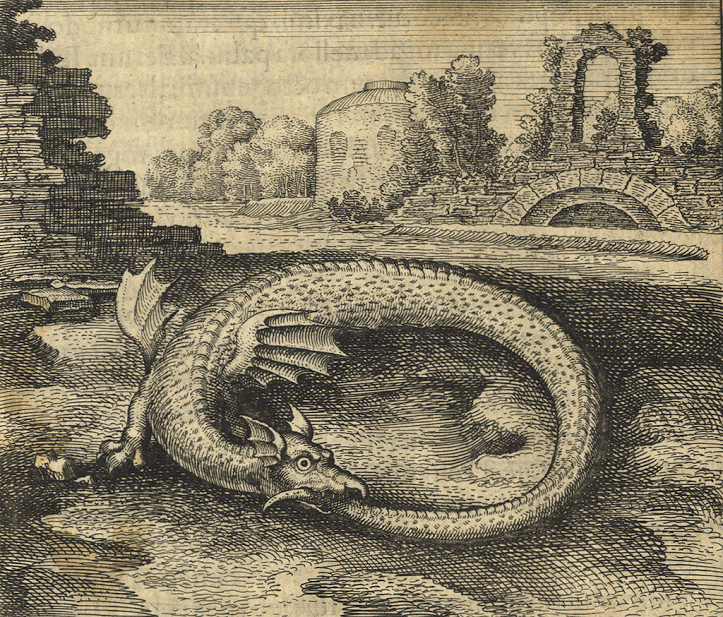Okay, now I've rather carefully discussed one example of \$$\mathcal{V}\$$-enriched profunctors, and rather sloppily discussed another. Now it's time to build the general framework that can handle both these examples.

We can define \$$\mathcal{V}\$$-enriched categories whenever \$$\mathcal{V}\$$ is a monoidal preorder: we did that way back in [Lecture 29](https://forum.azimuthproject.org/discussion/2121/lecture-29-chapter-2-enriched-categories/p1). We can also define \$$\mathcal{V}\$$-enriched functors whenever \$$\mathcal{V}\$$ is a monoidal preorder: we did that in [Lecture 31](https://forum.azimuthproject.org/discussion/2169/lecture-32-chapter-2-enriched-functors/p1). But to define \$$\mathcal{V}\$$-enriched profunctors, we need \$$\mathcal{V}\$$ to be a bit better. We can see why by comparing our examples.

Our first example involved \$$\mathcal{V} = \textbf{Bool}\$$. A **feasibility relation**

$\Phi : X \nrightarrow Y$

between preorders is a monotone function

$\Phi: X^{\text{op}} \times Y\to \mathbf{Bool} .$

We shall see that a feasibility relation is the same as a \$$\textbf{Bool}\$$-enriched profunctor.

Our second example involved \$$\mathcal{V} = \textbf{Cost}\$$. I said that a \$$\textbf{Cost}\$$-enriched profunctor

$\Phi : X \nrightarrow Y$

between \$$\mathbf{Cost}\$$-enriched categories is a \$$\textbf{Cost}\$$-enriched functor

$\Phi: X^{\text{op}} \times Y \to \mathbf{Cost}$

obeying some conditions. But I let you struggle to guess those conditions... without enough clues to make it easy!

To fit both our examples in a general framework, we start by considering an arbitrary monoidal preorder \$$\mathcal{V}\$$. \$$\mathcal{V}\$$-enriched profunctors will go between \$$\mathcal{V}\$$-enriched categories. So, let \$$\mathcal{X}\$$ and \$$\mathcal{Y}\$$ be \$$\mathcal{V}\$$-enriched categories. We want to make this definition:

**Tentative Definition.** A \$$\mathcal{V}\$$-enriched profunctor

$\Phi : \mathcal{X} \nrightarrow \mathcal{Y}$

is a \$$\mathcal{V}\$$-enriched functor

$\Phi: \mathcal{X}^{\text{op}} \times \mathcal{Y} \to \mathcal{V} .$

Notice that this handles our first example very well. But some questions appear in our second example - and indeed in general. For our tentative definition to make sense, we need three things:

1. We need \$$\mathcal{V}\$$ to itself be a \$$\mathcal{V}\$$-enriched category.

2. We need any two \$$\mathcal{V}\$$-enriched category to have a 'product', which is again a \$$\mathcal{V}\$$-enriched category.

3. We need any \$$\mathcal{V}\$$-enriched category to have an 'opposite', which is again a \$$\mathcal{V}\$$-enriched category.

Items 2 and 3 work fine whenever \$$\mathcal{V}\$$ is a commutative monoidal poset. We'll see why in [Lecture 62](https://forum.azimuthproject.org/discussion/2292/lecture-62-chapter-4-constructing-enriched-categories/p1).

Item 1 is trickier, and indeed it sounds rather scary. \$$\mathcal{V}\$$ began life as a humble monoidal preorder. Now we're wanting it to be _enriched in itself!_ Isn't that circular somehow?

Yes! But not in a bad way. Category theory often eats its own tail, like the mythical [ourobous](https://en.wikipedia.org/wiki/Ouroboros), and this is an example.To get \$$\mathcal{V}\$$ to become a \$$\mathcal{V}\$$-enriched category, we'll demand that it be 'closed'. For starters, let's assume it's a monoidal _poset_, just to avoid some technicalities.

**Definition.** A monoidal poset is **closed** if for all elements \$$x,y \in \mathcal{V}\$$ there is an element \$$x \multimap y \in \mathcal{V}\$$ such that

$x \otimes a \le y \text{ if and only if } a \le x \multimap y$

for all \$$a \in \mathcal{V}\$$.

This will let us make \$$\mathcal{V}\$$ into a \$$\mathcal{V}\$$-enriched category by setting \$$\mathcal{V}(x,y) = x \multimap y \$$. But first let's try to understand this concept a bit!

We can check that our friend \$$\mathbf{Bool}\$$ is closed. Remember, we are making it into a monoidal poset using 'and' as its binary operation: its full name is \$$\lbrace \text{true},\text{false}\rbrace, \wedge, \text{true})\$$. Then we can take \$$x \multimap y \$$ to be 'implication'. More precisely, we say \$$x \multimap y = \text{true}\$$ iff \$$x\$$ implies \$$y\$$. Even more precisely, we define:

$\text{true} \multimap \text{true} = \text{true}$
$\text{true} \multimap \text{false} = \text{false}$
$\text{false} \multimap \text{true} = \text{true}$
$\text{false} \multimap \text{false} = \text{true} .$

**Puzzle 188.** Show that with this definition of \$$\multimap\$$ for \$$\mathbf{Bool}\$$ we have

$a \wedge x \le y \text{ if and only if } a \le x \multimap y$

for all \$$a,x,y \in \mathbf{Bool}\$$.

We can also check that our friend \$$\mathbf{Cost}\$$ is closed! Remember, we are making it into a monoidal poset using \$$+\$$ as its binary operation: its full name is \$$[0,\infty], \ge, +, 0)\$$. Then we can define \$$x \multimap y \$$ to be 'subtraction'. More precisely, we define \$$x \multimap y\$$ to be \$$y - x\$$ if \$$y \ge x\$$, and \$$0\$$ otherwise.

**Puzzle 189.** Show that with this definition of \$$\multimap\$$ for \$$\mathbf{Cost}\$$ we have

$a + x \le y \text{ if and only if } a \le x \multimap y .$

But beware. [We have defined the ordering on \$$\mathbf{Cost}\$$ to be the _opposite_ of the usual ordering of numbers in \$$[0,\infty]\$$](https://forum.azimuthproject.org/discussion/2128/lecture-31-chapter-2-lawvere-metric-spaces/p1). So, \$$\le\$$ above means the _opposite_ of what you might expect!Next, two more tricky puzzles. Next time I'll show you in general how a closed monoidal poset \$$\mathcal{V}\$$ becomes a \$$\mathcal{V}\$$-enriched category. But to appreciate this, it may help to try some examples first:

**Puzzle 190.** What does it mean, exactly, to make \$$\mathbf{Bool}\$$ into a \$$\mathbf{Bool}\$$-enriched category? Can you see how to do this by defining

$\mathbf{Bool}(x,y) = x \multimap y$

for all \$$x,y \in \mathbf{Bool}\$$, where \$$\multimap\$$ is defined to be 'implication' as above?

**Puzzle 191.** What does it mean, exactly, to make \$$\mathbf{Cost}\$$ into a \$$\mathbf{Cost}\$$-enriched category? Can you see how to do this by defining

$\mathbf{Cost}(x,y) = x \multimap y$

for all \$$x,y \in \mathbf{Cost}\$$, where \$$\multimap\$$ is defined to be 'subtraction' as above?

Note: for Puzzle 190 you might be tempted to say "a \$$\mathbf{Bool}\$$-enriched category is just a preorder, so I'll use that fact here". However, you may learn more if you go back to the [general definition of enriched category](https://forum.azimuthproject.org/discussion/2121/lecture-29-chapter-2-enriched-categories/p1) and use that! The reason is that we're trying to understand some general things by thinking about two examples.

**Puzzle 192.** The definition of 'closed' above is an example of a very important concept we keep seeing in this course. What is it? Restate the definition of closed monoidal poset in a more elegant, but equivalent, way using this concept.

**[To read other lectures go here.](http://www.azimuthproject.org/azimuth/show/Applied+Category+Theory#Chapter_4)**